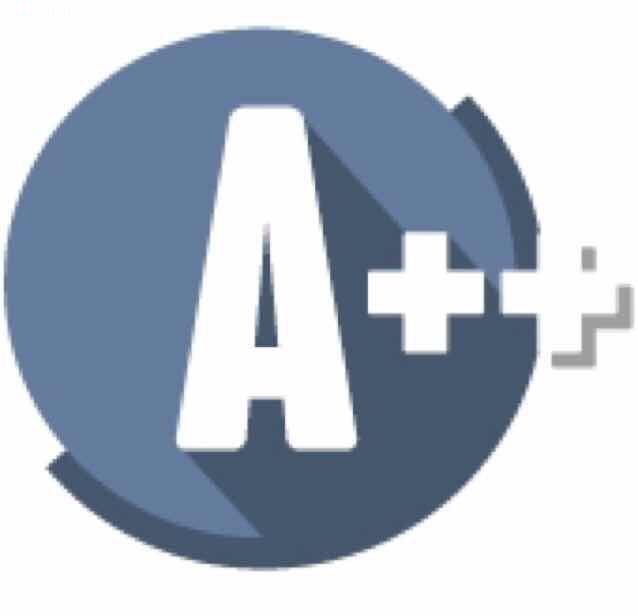## Interviews

#### String Pattern MatchingThe matching should cover the entire input string (not partial).
The function prototype should be:
bool isMatch(String str, String pattern)
Some examples:
isMatch("aa","a") → false
isMatch("aa","aa") → true
isMatch("aaa","aa") → false
isMatch("aa","a{1,3}") → true
isMatch("aaa","a{1,3}") → false
isMatch("ab","a{1,3}b{1,3}") → true
isMatch("abc","a{1,3}b{1,3}c") → true
isMatch("abbc","a{1,3}b{1,2}c") → false
isMatch("acbac","a{1,3}b{1,3}c") → false
isMatch("abcc","a{1,3}b{1,3}cc{1,3}") → true
In pattern string, a char followed by {lower, upper} means that the char occur lower to upper(exclusive) times. e.g. a{1, 3} -> a occurs 1 or 2 times.

``````
public boolean match(String str, String pattern) {
int occurLower = 0, occurUpper = 0;
char prev = '\0';
int i = 0, j = 0;

while(j < pattern.length()) {
char c = i == str.length() ? '\0': str.charAt(i);
if (occurUpper == 0 || prev == pattern.charAt(j) || (c != prev && occurLower <= 0)) {
String range = j + 1 < pattern.length() && pattern.charAt(j + 1) == '{' ?
pattern.substring(j + 2, pattern.indexOf('}', j + 2)): "";
int[] r = getRange(range);
occurLower = prev == pattern.charAt(j) ? occurLower + r : r;
occurUpper = prev == pattern.charAt(j) ? occurUpper + r : r;
prev = pattern.charAt(j);
j = range.isEmpty() ? j + 1: pattern.indexOf('}', j + 2) + 1;
}
if (c == prev) {
occurLower--;
occurUpper--;
i++;
} else if (occurLower > 0) {
return false;
}
}
while(i < str.length()) {
if(str.charAt(i) != prev || occurUpper == 0) return false;
else {
occurUpper--;
i++;
}
}
return true;
}

private int[] getRange(String b) {
if(b.isEmpty()) return new int[]{1,1};
int comma = b.indexOf(',');
return new int[]{
Integer.parseInt(b.substring(0, comma)),
Integer.parseInt(b.substring(comma + 1)) - 1
};
}
``````